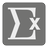Simplification
Challenging
English FR RU

"I do believe in simplicity. It is astonishing as well as sad, how many trivial affairs even the wisest thinks he must attend to in a day; how singular an affair he thinks he must omit. When the mathematician would solve a difficult problem, he first frees the equation of all incumbrances, and reduces it to its simplest terms. So simplify the problem of life, distinguish the necessary and the real. Probe the earth to see where your main roots run."
-― Henry David Thoreau

In mathematics, a polynomial is an expression consisting of variables (or indeterminates) and coefficients, that involves only the operations of addition, subtraction, multiplication, and non-negative integer exponents.

You are given a mathematical expression with brackets and one variable as a string. The expression contains "x", brackets and the following operators: + - * . You should convert this to a Polynomial. The power of "x" should be represented by "**".

The final form of the polynomial should be in the following format:

```     CN*x**n+CN1*x**(n-1)+...+C1*x+C0
```

where
С N , С N1 ...С 1 , С 0 - constants, integers.
if C = 0, then don't show this term (ex. 2*x**2+3). The only exception is alone zero (ex. "x-x" == "0").
if C = 1, then don't show C. (ex. x**2+2*x). Only if it's not С 0 .
if C = -1, then don't show C, only "-". (ex. x*x-x). Only if it's not С 0 .
if power of x is 1, then don't show the power (ex. 5*x). Terms must be in descending order, sorted by the power of the variable.

Input: An expression as a string (unicode).

Output: A polynomial as a string without whitespaces.

Example:

```simplify("(x-1)*(x+1)") == "x**2-1"
simplify("(x+1)*(x+1)") == "x**2+2*x+1"
simplify("(x+3)*x*2-x*x") == "x**2+6*x"
simplify("x+x*x+x*x*x") == "x**3+x**2+x"
simplify("(2*x+3)*2-x+x*x*x*x") == "x**4+3*x+6"
simplify("x*x-(x-1)*(x+1)-1") == "0"
simplify("5-5-x") == "-x"
simplify("x*x*x-x*x*x-1") == "-1"
```

How it is used: It's a very useful function for mathematical software or finishing your math homework. Time to wrap your head around it!

Precondition:
0 < len(expr)

Settings
Code:
CheckiO Extensions

CheckiO Extensions allow you to use local files to solve missions. More info in a blog post.

In order to install CheckiO client you'll need installed Python (version at least 3.8)

Install CheckiO Client first:

`pip3 install checkio_client`

`checkio --domain=py config --key=`

Sync solutions into your local folder

`checkio sync`

(in beta testing) Launch local server so your browser can use it and sync solution between local file end extension on the fly. (doesn't work for safari)

`checkio serv -d`

Alternatevly, you can install Chrome extension or FF addon

`checkio install-plugin`
`checkio install-plugin --ff`
`checkio install-plugin --chromium`

Read more here about other functionality that the checkio client provides. Feel free to submit an issue in case of any difficulties.

Sync...